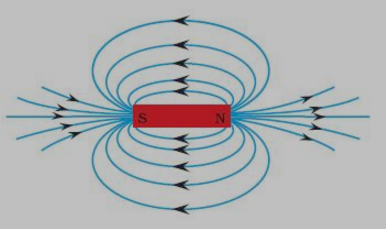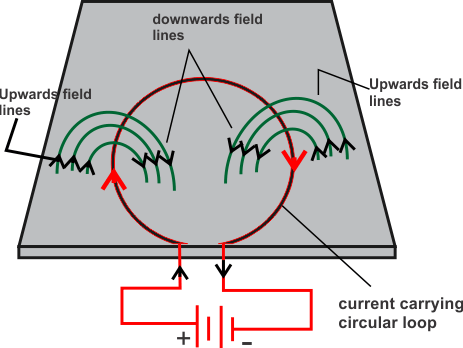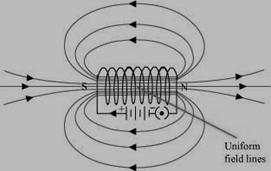# Class 10 Magnetic effects of current NCERT Solutions (in text questions)

Page No: 224

Question 1 Why does a compass needle get deflected when brought near a bar magnet?
Compass needle is a small permanent magnet. That is why when compass needle is brought near a bar magnet, its magnetic field lines interact with that of the bar magnet. This is the reason why a compass needle gets deflected when it is bought near a bar magnet.
Extra thought on what exactly is happening here:-
We use compass to trace the magnetic field lines of a bar.  The needle of a compass is itself a permanent magnet and the north indicator (generally red in color) of the compass is a magnetic north pole. The north pole of a magnet will tend to line up with the magnetic field, so a compass needle bought under the influence of a bar magnet will rotate until it lines up with the magnetic field.

Page No: 228

Question 1 Draw magnetic field lines around a bar magnet.
The magnetic field lines of a bar magnet form closed lines. The direction of magnetic field lines is taken to be emerging from the North Pole and terminating at the South Pole.
As can be seen from the figure, the strongest external magnetic fields are near the poles.Please note that the magnetic field is strongest inside the magnetic material.
Question 2 List the properties of magnetic lines of force.
the properties of magnetic lines of force are as follows.
1. All field lines are closed curves.
2. Outside the magnet field lines emerge from North Pole and merge at South Pole.
3. Inside a magnet, the direction of field lines is from South Pole to its north pole.
4. Field lines never intersect each other.
5. Field lines are closed together near the poles and spread out away from them.
6. The field is stronger where the field lines are more closely spaced. So, the field is stronger near the poles then at other points.
Extra point- Magnetic field lines give the direction of the magnetic field at any given point
Question 3 Why don't two magnetic lines of force intersect each other?
The two magnetic field lines do not intersect each other because If two lines were to intersect each other, then a compass needle placed at the point of interaction would point in two different directions which is not possible.

Page No: 229

Question 1 Consider a circular loop of wire lying in the plane of the table. Let the current pass through the loop clockwise. Apply the right-hand rule to find out the direction of the magnetic field inside and outside the loop.
It is given in the question that circular loop is lying on the table and we have connected this loop to some battery or power supply so that current is flowing in the loop in clockwise direction.
Inside the loop in = Magnetic field lines would pierce inside the table
Outside the loop =Magnetic field lines would appear to emerge out from the table
For downward direction of current flowing in the circular loop, the direction of magnetic field lines will be as if they are emerging from the table outside the loop and merging in the table inside the loop. Similarly, for upward direction of current flowing in the circular loop, the direction of magnetic field lines will be as if they are emerging from the table outside the loop and merging in the table inside the loop, as shown in the given figure.Note: - right hand thumb rule can be applied to find the direction of magnetic field lines in respective directions of current flow. Here note that in one half of circular loop current is flowing in upwards direction and in another half it is flowing in downwards direction. So I insist try to find the field accordingly.
Question 2 The magnetic field in a given region is uniform. Draw a diagram to represent it.
The magnetic field lines are uniform inside a current carrying long straight solenoidPage No: 230

Question 3 Choose the correct option.
The magnetic field inside a long straight solenoid-carrying current
(a) is zero
(b) decreases as we move towards its end
(c) increases as we move towards its end
(d) is the same at all points
Answer (d) is the same at all points
The magnetic field inside a long, straight, current carrying solenoid is uniform. It is same at all points inside the current carrying solenoid.

Page No: 231

Question 1 Which of the following property of a proton can change while it moves freely in a magnetic field? (There may be more than one correct answer.)
(a) Mass
(b) speed
(c) velocity
(d) momentum
Answer (c) velocity and (d) momentum
When a proton enters in a region of magnetic field, it experiences a magnetic force. The magnetic force, acting perpendicular to the velocity of the particle, will cause circular motion. Hence, velocity and momentum of proton changes when it moves in a magnetic field.
Extra point- Velocity is a vector quantity thus it changes with the change in direction. This is a case of uniform circular motion and speed of the charged particle in the magnetic field remains constant.

Page No: 232

Question 2 In Activity 13.7 (page: 230), how do we think the displacement of rod AB will be affected if (i) current in rod AB is increased: (ii) a stronger horse-shoe magnet is used: and (iii) length of the rod AB is increased?
Concept: - A force is experienced by a current carrying conductor placed in a magnetic field. The magnitude of force increases with the amount of current, strength of the magnetic field and with the change in the length of the conductor. Hence the magnetic force exerted on rod increases.
Deflection of rod in a magnetic field increases if,
(i) If the current in the rod is increased then rod will be deflected with greater force.
(ii) If a stronger horse-shoe magnet is used then also rod will be deflected with greater force due to the increase in magnetic field.
(iii) If the length of the rod AB is increased.
Question 3 A positively-charged particles (alpha-particle) projected towards west is deflected towards north by a magnetic field. The direction of magnetic field is
(a) towards south (b) towards east
(c) downward (d) upward
Answer (d) upward. The direction of the magnetic field can be determined by the Fleming’s left hand rule.2
Extra point:- According to Fleming’s left hand rule, if we arrange the thumb , the middle finger and the forefinger of the left hand at right angles to each other, then thumb points towards the direction of magnetic force, middle finger gives the direction of current and the forefinger points in the direction of magnetic field.
Since the direction of positively charged alpha particle is towards west, the direction of current will be the same that is towards the west. Again the direction of magnetic force would be towards the North. Hence, according to Fleming’s left hand rule, the direction of magnetic field will be along upwards direction.

Page No: 233

Question 1 State Fleming's left-hand rule.
According to Fleming’s left hand rule, if we arrange the thumb, the middle finger and the forefinger of the left hand at right angles to each other, then thumb points towards the direction of magnetic force, middle finger gives the direction of current and the forefinger points in the direction of magnetic field.

Question 2 What is the principle of an electric motor?
The principle of an electric motor is based on the magnetic effect of electric current. A current-carrying loop when placed in a magnetic field experiences a force and begins to rotate. The direction of rotation of the loop is according to the Fleming’s left-hand rule.

Question 3 What is the role of the split ring in an electric motor?
The split ring in the electric motor acts as a commutator that reverses the direction of current flowing through the coil after every half rotation of the coil. Due to this reversal of the current the coil continues to rotate in the same direction.

Page No: 236

Question 1 Explain different ways to induce current in a coil.
The different ways to induce current in a coil are as follows:
1. If a magnet is moved relative to a coil, then an electric current is induced in the coil.
2. If a coil is being moved towards or away from a magnet then an electric current is induced in the coil.

Page No: 237

Question 1. State the principle of an electric generator.
Electric generator works on the principle of electromagnetic induction When a coil is rotated in magnetic field electricity can be generated.

Question 2 Name some sources of direct current.
some sources of direct current are cell or battery, DC generator, etc.

Question 3 Which sources produce alternating current?
AC generators, power plants, etc., produce alternating current.

Question 4 Choose the correct option.
A rectangular coil of copper wires is rotated in a magnetic field. The direction of the induced current changes once in each
(a) two revolutions (b) one revolution
(c) half revolution (d) one-fourth revolution
Explanation – When a rectangular coil of copper wire is rotated in a magnetic field, the direction of the current induced in the coil changes once in each half revolution. As a result, the direction of current in the coil remains the same. This happens because the commutator reverses the direction of current flowing through the coil after each half rotation of the coil.

Page No: 238

Question 1. Name two safety measures commonly used in electric circuits and appliances.
Two safety measures commonly used in electric circuits and appliances are
1. Each circuit must be connected with an electric fuse. This prevents flow of excess flow of current in the circuit. When the current passing through the circuit exceeds a maximum limit of the fuse used then the fuse melts and flow of current in the circuit is stopped. This is how fuse protects appliances in the circuit.
2. Earthing is a must if you want to prevent electric shocks when you use electric appliances. Any leakage of current in electric appliance is transferred to ground and this way we do not get shock.

Question 2 An electric oven of 2KW is operated in domestic current (220V) that has current rating of 5A. What result do you expect? Explain.
Current drawn by the electric oven can be obtained by the expression,
P = VI
So, Current $I=\frac{P}{V}$ Power of the oven, P = 2 kW = 2000 W
Voltage supplied, V = 220 V
I = 2000/220 = 9.09 A
Hence, the current drawn by the electric oven is 9.09 A, which exceeds the safe limit of the circuit. Fuse element of the electric fuse will melt and break the circuit.

Question 3 What precaution should be taken to avoid the overloading of domestic electric circuits?

The precautions that should be taken to avoid the overloading of domestic circuits are as follows:
1. Too many appliances should not be connected to a single socket.
2. Too many appliances should not be used at the same time.
3. Faulty appliances should not be connected in the circuit.
4. Fuse should be connected in the circuit.